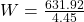## From the gravitational law, calculate the weight W (gravitational force with respect to the earth) of a 70 kg spacecraft traveling in a circ

Question

From the gravitational law, calculate the weight W (gravitational force with respect to the earth) of a 70 kg spacecraft traveling in a circular orbit 275 km above the earth’s surface. Express W in Newtons and pounds.

in progress 0
2 months 2021-07-29T20:08:11+00:00 1 Answers 1 views 0

The  value in Newton is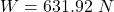The  value in pounds is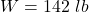Explanation:

From the question we are told that

The  mass of the spacecraft is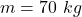The distance above  the earth is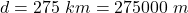Generally the gravitational force with respect to the earth is mathematically represented as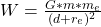Here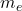is the mass of earth with value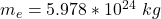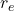is the radius of the earth with value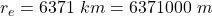G is the gravitational constant with value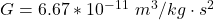So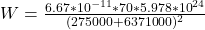Converting to  pounds